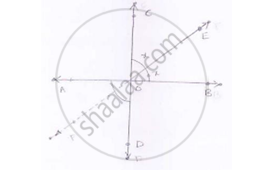# Prove-that-bisectors-pair-vertically-opposite-angles-are-same-straight-line - Mathematics

Prove that the bisectors of a pair of vertically opposite angles are in the same straight line.

#### Solution

Given,

Lines  AOB and COD intersect at point O such that

∠AOC = ∠BOD

Also OE is the bisector ∠ADC and OF is the bisector ∠BOD

To prove: EOF is a straight line vertically opposite angles is equal

∠AOD = ∠BOC = 5x        .......(1)

Also ∠AOC + ∠BOD

⇒ 2∠AOE = 2∠DOF          .......(2)

Sum of the angles around a point is 360°

⇒ 2∠AOD + 2∠AOE + 2∠DOF = 360°

⇒∠AOD + ∠AOF + ∠DOF = 180°

From this we conclude that EOF is a straight line.Given that :- AB and CD intersect each other at O

OE bisects  ∠COB

To prove: ∠AOF = ∠DOF

Proof: OE bisects ∠COB

∠COE = ∠EOB = x

Vertically opposite angles are equal

∠BOE = ∠AOF = x         .......(1)

∠COE = ∠DOF = x          .......(2)

From (1) and (2)

∠AOF = ∠DOF = x

Concept: Concept of Parallel Lines
Is there an error in this question or solution?

#### APPEARS IN

RD Sharma Mathematics for Class 9
Chapter 10 Lines and Angles
Exercise 10.3 | Q 12 | Page 23